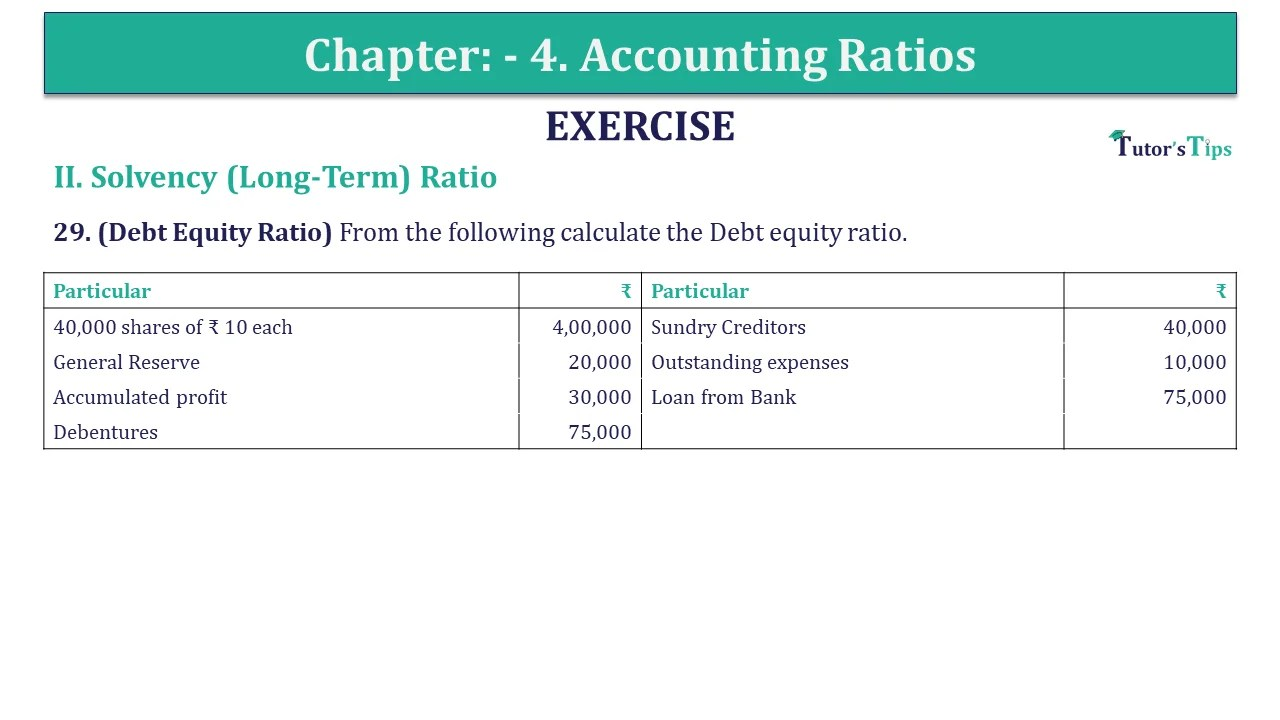# Question 29 Chapter 4 of +2-B – USHA Publication 12 ClassQ-29- CH-4 Book 2 - Usha Pub. +2 Book 2020 - Solution

Question 29 Chapter 4 of +2-B

II. Solvency (Long-Term) Ratio

29. (Debt Equity Ratio) From the following calculate the Debt equity ratio.

 Particular ₹ Particular ₹ 40,000 shares of ₹ 10 each 4,00,000 Sundry Creditors 40,000 General Reserve 20,000 Outstanding expenses 10,000 Accumulated profit 30,000 Loan from Bank 75,000 Debentures 75,000

## The solution of Question 29 Chapter 4 of +2-B: –

 Debt Equity Ratio = Debt Shareholders Funds
 Debt = Debentures + Loan from Bank = ₹ 75,000 + ₹ 75,000 = ₹ 1,50,000 Shareholders Funds = Share capital + General Reserve + Accumulated Profit = ₹ 4,00,000 + ₹ 20,000 + ₹ 30,000 = ₹ 4,50,000
 Debt Equity Ratio = ₹ 1,50,000 ₹ 4,50,000 = 0.33: 1

What are Liquidity Ratios – Formulas and Examples

Comment if you have any question.

Also, Check out the solved question of previous Chapters: –